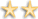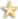Guaranteed review within the next five business days. (One Time Fee - \$39.99)

1 - 22

Multiplication.com
Offers sections for teachers, students, and parents. Includes activities, worksheets, and games to help children learn the times tables.A+ Math
Offers flash card maker, worksheets, homework helper, and math games.Algebra Homework Help
Offers interactive tools for learning algebra, homework help, word problems, forum and shopping for books, toys and software.Cool Math.com
Featuring links to several of their sister sites, CoolMath has many resources to help out parent, teachers, and students.Figure This
Math challenges for middle school children and their families. Each contains a description of math involved, how math is used in the real world, hints on getting started, fun facts. Available in English and Spanish.Hooda Math
Offers math games, math worksheets, math tutorials, and math movies for kids.Math Goodies
Math resources for students and teachers. Interactive math lessons, calculators, homework help, puzzles and a newsletter.Math Guide
Includes puzzles, projects, and lessons. Offers separate sections for parents, students, and teachers.Math K-Plus
Kids kindergarten through fourth grade learn problem solving skills by math practice: Addition, Subtraction, Division, and Multiplication.Math2.org
Searchable site with math reference tables and links to related resources.Maths Dictionary for Kids
Contains entries arranged alphabetically explaining key math terms and concepts in a simple, straightforward manner. Besides giving definitions, each topic has an interactive activity that demonstrates its use and significance.Online Chart Tool
Online chart and graph builder. Charts include: bar, pie, line, xy, radar, meter, plot, xy, area and bubble. In English and Dutch.Quick Math
Automatically solves problems in algebra and calculus, solve an equation or inequalities, make graphs, get percentages, or get commands for the arithmetic manipulation of matrices.S.O.S. Mathematics
Includes information about algebra, trigonometry, calculus, differential equations, and more.Color Math Pink
Membership only site offers tutoring and other math help for girls.Free Math Worksheets
Large number of free math worksheets for teachers and students sorted by difficulty.Learn With Math Games
Teacher-approved math games to motivate and teach math.Math for Morons Like Us
Learn algebra, geometry, and calculus. Also offers message boards and sample quizes.Mathletics
Find out how math is used in baseball, football, bowling, and basketball.Mathnook
Free math games that target a variety of math skills from counting all the up to multiplying positive and negative integers.TeachMeWell
Provides online math Flash tutorials, practice lessons, timed tests, games and printable worksheets. Results are logged and graded to help your child improve their math skills.Uncle Math
Provides configurable platform to practice math table, whole number, decimal and fraction related problems. It gives the experience of practicing problems like solving it on paper.